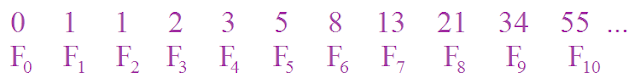## Pages

### Fibonacci numbers and continued fractionsFibonacci is probably the most well known sequence in mathematics. Today, we will see how Fibonacci numbers can be used to construct beautiful patterns called continued fractions.

Fibonacci numbers are the following $$0, ~1, ~1, ~2, ~3, ~5, ~8, ~13, ~21, ~34, ~55, ~89, ~144, \dots$$ We can easily see the pattern of this number sequence. Each Fibonacci number is equal to the sum of the previous two numbers.

Mathematically, we can write the formula as follows $$F_0 = 0, ~F_1 = 1, ~~F_n = F_{n-1} + F_{n-2}$$Now, let us consider the Fibonacci fractions $\frac{F_n}{F_{n-1}}$.

First, $$\frac{F_2}{F_1} = \frac{1}{1}$$
Next, $$\frac{F_3}{F_2} = \frac{2}{1} = 1 + \frac{1}{1}$$
$$\frac{F_4}{F_3} = \frac{3}{2} = 1 + \frac{1}{2} = 1 + \cfrac{1}{\frac{2}{1}} = 1 + \cfrac{1}{1 + \cfrac{1}{1}}$$
$$\frac{F_5}{F_4} = \frac{5}{3} = 1 + \frac{2}{3} = 1 + \cfrac{1}{\frac{3}{2}} = 1 + \cfrac{1}{1 + \cfrac{1}{1 + \cfrac{1}{1}}}$$
That is so cool, isn't it?!
$$\frac{F_6}{F_5} = \frac{8}{5} = 1 + \frac{3}{5} = 1 + \cfrac{1}{\frac{5}{3}} = 1 + \cfrac{1}{\frac{F_5}{F_4}} = 1 + \cfrac{1}{1 + \cfrac{1}{1 + \cfrac{1}{1 + \cfrac{1}{1}}}}$$
So we can follow the pattern and derive the following beautiful continued fractions
$$\frac{F_7}{F_6} = \frac{13}{8} = 1 + \cfrac{1}{\frac{F_6}{F_5}} = 1 + \cfrac{1}{1 + \cfrac{1}{1 + \cfrac{1}{1 + \cfrac{1}{1 + \cfrac{1}{1}}}}}$$
$$\frac{F_8}{F_7} = \frac{21}{13} = 1 + \cfrac{1}{\frac{F_7}{F_6}} = 1 + \cfrac{1}{1 + \cfrac{1}{1 + \cfrac{1}{1 + \cfrac{1}{1 + \cfrac{1}{1 + \cfrac{1}{1}}}}}}$$

Let us stop here for now. You can read more about Fibonacci numbers here. Hope to see you again next time.